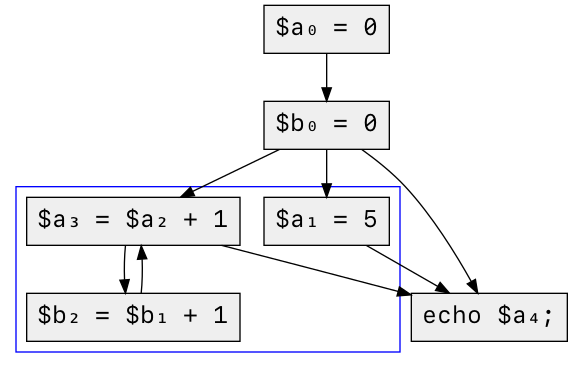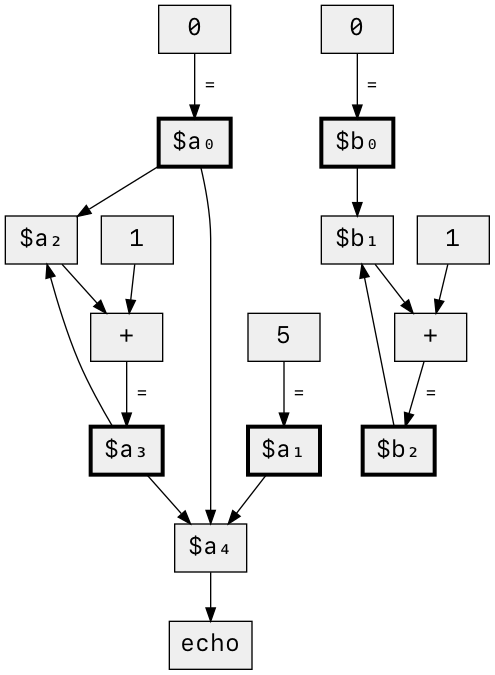# Better Unused Variable Detection in Psalm 4

October 21, 2020 by Matt Brown - 3 minute read

This is part of a series of articles about the new features of Psalm 4.

The new version of Psalm comes with massively-improved unused variable detection. To understand why the new system is better, we're going to get a bit theoretical.

## The status quo

Psalm added unused variable detection three years ago, modelled on the unused variable detection used by PhpStorm & TypeScript. It was a basic mark-and-sweep system: for any given assignment to a variable, is that assignment used anywhere?

Consider this dummy code:

``````function foo() : void {
\$b = \$a = 0;

while (first_condition()) {
if (second_condition() && third_condition()) {
\$a = 5;
break;
}

if (fourth_condition()) {
continue;
}

\$a = \$a + 1;
\$b = \$b + 1;
}

echo \$a;
}
``````

Looking at the above we can see that all assignments to `\$a` inform the value passed to `echo`, but the same is not true of `\$b` – its value is used on the right-hand-side of `\$b = \$b + 1`, but that assignment doesn't end up anywhere useful. This useless usage is enough to satisfy the mark-and-sweep approach (which thus marks `\$b` as used), but it doesn't satisfy us.

## The solution

To solve this problem, we'll need to explore the imaginary web of connections that underpins Psalm's type inference: the control-flow graph.

A control-flow graph shows how blocks of code – `if` statements, ternaries, loops, etc – inform the control-flow of a program. The code above translates to this control-flow graph below (we add a subscript to every occurrence of `\$a` and `\$b` to help distinguish their usage).Since we only care about variables and their eventual usage, we can simplify that graph.From this control-flow graph we can derive something even more useful to us: a data-flow graph. Data-flow graphs show how individual expressions relate to one another, and if you can generate a control-flow graph then it's trivial to generate a corresponding data-flow graph. For the above code, the data-flow graph looks like this (assignment nodes have an extra-thick outline):With this data-flow graph it’s easy to see that all assignments to `\$a` flow into an eventual `echo` value, while none of the assignments to `\$b` do.

This is how Psalm's improved unused variable detection operates – it constructs a data-flow graph for every function and file, then does a simple graph traversal to verify that every assignment is used somewhere.

## Fringe benefits

Psalm already generated data-flow graphs to power its taint analysis, so I was able to reuse most of that logic for unused variable detection. The extra attention on data-flow graph generation has also helped improve the accuracy of Psalm’s existing taint analysis, so if you’re curious to try that out there’s never been a better time.

Another bonus: undefined variable detection is now much more closely tied to Psalm’s type inference than it was before, so for each false-positive `UndefinedVariable` bug there's usually an equivalent false-negative type inference bug, where a fix for one is also a fix for the other (ping me on Twitter if you want more details).

## Try it out

Psalm highlights the unused code in the interactive block below. Click the green button to make the assignments to `\$b` disappear!

``````<?php // fix

function foo() : void {
\$b = \$a = 0;

while (rand(0, 1)) {
if (rand(0, 1)) {
\$a = 5;
break;
}

\$a = \$a + 1;
\$b = \$b + 1;
}

echo \$a;
}
``````

You can remove all unused variables in your own projects automatically with

``````vendor/bin/psalm --alter --issues=UnusedVariable
``````